# HSSlive: Plus One & Plus Two Notes & Solutions for Kerala State Board

## Plus Two Maths Chapter 10 Vector Algebra Notes PDF- Download Class 12 Maths Chapter 10 Vector Algebra Solutions PDFPlus Two Maths Chapter 10 Vector Algebra Notes PDF Download Class 12 Maths Chapter 10 Vector Algebra Solutions PDF

Plus Two Maths Chapter 10 Vector Algebra Notes: Students can download the Plus Two Maths Chapter 10 Vector Algebra Notes PDF from our website for free. These Plus Two Maths Chapter 10 Vector Algebra Notes will help students to prepare efficiently for the upcoming SCERT board exams. We have also uploaded Plus Two notes for all subjects from this page.

## Plus Two Maths Chapter 10 Vector Algebra Notes

Plus Two Maths Chapter 10 Vector Algebra Chapter Wise Notes PDF and Key Points for Class 12 Maths Chapter 10 Vector Algebra Notes are designed by expert teachers from the latest edition of SCERT books to get good marks in board exams. SCERT Plus Two Maths Chapter 10 Vector Algebra Notes contains all chapters Of Maths Chapter 10 Vector Algebra. Here we have given SCERT Maths Chapter 10 Vector Algebra Notes Class 12. We recommend you to study SCERT Solutions for Class 12 Maths Chapter 10 Vector Algebra.
 Board Kerala Board Class Plus Two Subject Maths Chapter Chapter 10 Vector Algebra Format PDF Provider hsslive.co.in

## How to download Plus Two Maths Chapter 10 Vector Algebra Notes PDF?

1. Visit the website of hsslive.
2. Search for Notes.
3. Then Look for Plus Two Notes.
5. Download the notes for Maths Chapter 10 Vector Algebra using the links provided in the article.

## Plus Two Maths Chapter 10 Vector Algebra Notes Chapter Wise PDF Download

Attached is a detailed study notes of all the subjects based on the of ​​Plus Two Maths Chapter 10 Vector Algebra for the students studying in the Higher Secondary section of Kerala. Students can understand these lessons better without going to school and get better marks in the exams. Study notes of each lesson can be downloaded and used by everyone.

Introduction
Physical quantities we deal are of two types, one that can be specified using a single real number which gives its magnitude and the other which involves the idea of direction as well as magnitude. The first type is called scalar quantity and the second is vector quantity. In this chapter we analyses the basic concepts about vectors, various operations, and their algebraic and geometrical properties.

I. Types of vectors

1. Equal Vectors: Vectors having same magnitude and direction regardless of the positions of their initial points.
2. Collinear Vectors: Vectors which are parallel to the same line, irrespective of their magnitude and direction.
3. Like and Unlike Vectors: Collinear vectors having same direction are like vectors and opposite direction are unlike vectors.
4. Unit Vectors: Vectors with magnitude unity.

II. Component form of a vector
Let i, j, k be the unit vectors along the x-axis, y-axis, z-axis respectively. The point P(x, y, z) be a point in space. Then the position vector of the point P can be expressed in component form as1. If li + mj + nk is unit vector, then l,m,n are direction cosines along the vector.
2. If P (a, b, c) is a point on space, then a, b, c are direction ratios andare direction cosines along the vector OP¯¯¯¯¯¯¯¯.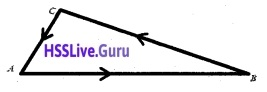AB¯¯¯¯¯¯¯¯+BC¯¯¯¯¯¯¯¯+CA¯¯¯¯¯¯¯¯=0¯¯¯ is known as triangle law of vector addition.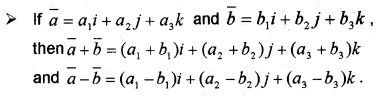IV. Multiplication of a vector by a scalar
Let a¯ = a1i + a2j + a3k be a vector and λ be a scalar. Then the product of the vector a¯ by a scalar is denoted by λa¯ and the new vector formed has a magnitude λ|a¯|.
λa¯ = λa1i + λa2j + λa3k

V. Vector joining two points
If P(a1, a2, a3) and Q(b1, b2, b3) are two points, then the vector joining P and Q is the vector PQ¯¯¯¯¯¯¯¯.
ie: PQ¯¯¯¯¯¯¯¯ = (b1 – a1)i + (b2 – a2)j + (b3 – a3)k

VI. Section Formula
If a¯ and b¯ be the position vectors of the points A and B respectively, then the position vector of the point P which divides AB in the ratio l:mVII. Dot (Scalar) Product of vectors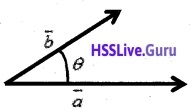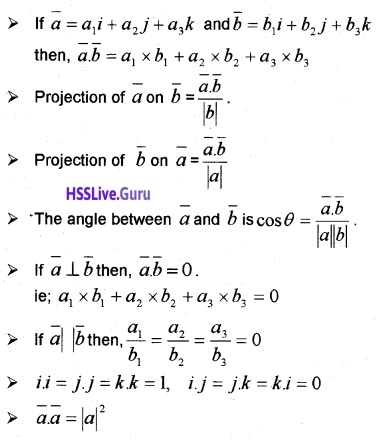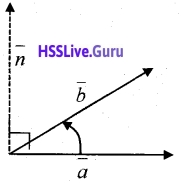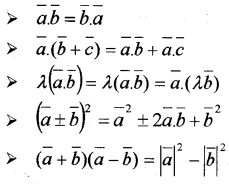VIII. Cross (vector) Product of Vectors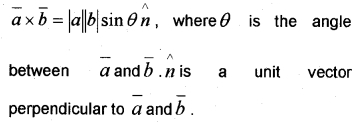• a¯×b¯ is a vector perpendicular to a¯ and b¯.
• |a¯×b¯| gives the area of a parallelogram with adjacent sides a¯ and b¯.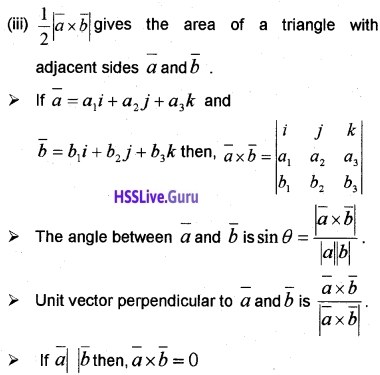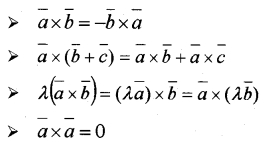• i × i = j × j = k × k = 0,
• i × j = k, j × k = i, k × i = j
• j × i = -k, k × j = -i, i × k = -j

IX. Box (Scalar Triple) Product of VectorsProperties:
1. Since b¯×c¯ is a vector, [a¯b¯c¯] is a scalar quantity.

2. |[a¯b¯c¯]| is the volume of the parallelopiped with a adjacent sides vector a¯,b¯,c¯.

3. If a¯ = a1i + a2j + a3k; b¯ = b1i + b2j + b3k and c¯ = c1i + c2j + c3k, then4. if a¯,b¯,c¯ be any three vectors, then [a¯b¯c¯] = [b¯c¯a¯]=[c¯a¯b¯] (cyclic permutation of three vectors does not change the value of the scalar triple product).

5. In scalar triple product, the dot and cross can be interchanged.ie,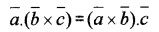6. If any two vectors are interchanged the sign of box product is changed but magnitude remains the same.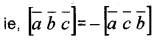7. If any two vectors are equal or proportional then the value of box product is zero.

8. Three vectors a¯,b¯,c¯ are coplanar if and only if [a¯b¯c¯] = 0.

## Highlights of Plus Two Maths Chapter 10 Vector Algebra Notes

Some of the advantages of studying from these Plus Two Maths Chapter 10 Vector Algebra notes:
• These notes are prepared by our highly experienced exam experts and are purely based on the latest Plus Two Maths Chapter 10 Vector Algebra syllabus as prescribed by the SCERT board.
• These Plus Two Maths Chapter 10 Vector Algebra notes cover all the important concepts, facts, equations and formulas included in the syllabus.
• These Maths Chapter 10 Vector Algebra notes are designed in a precise manner so as to provide all the important illustrations making it much easier for students to grasp important concepts crucial for the examination perspective.